# How to Calculate and Solve for Gravitational Force | The Calculator Encyclopedia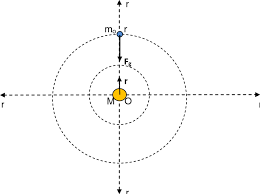The image above represents the gravitational force.

To compute the gravitational force of a field, three parameters are needed and this parameters are mass (m1), mass (m2) and radius between the masses (R).

The formula for calculating the gravitational force:

F = Gm1m2 /

Where;
F = Gravitational force
m1 = Mass 1
m2 = Mass 2
r = Radius between the masses

Let’s solve an example;
Find the gravitational force of a field when the mass 1 is 8 cm, mass 2 is 10 cm and the radius between masses is 14 cm.

This implies that;
m1 = Mass 1 = 8 cm
m2 = Mass 2 = 10 cm
r = Radius between the masses = 14 cm

F = Gm1m2 /
F = (6.67 x 10-11 x 8 x 10) / 196
F = 5.336e-9 / 196
F = 2.722e-11

Therefore, the gravitational force is 2.722e-11 Newton (N).

Nickzom Calculator – The Calculator Encyclopedia is capable of calculating the gravitational force.

To get the answer and workings of the gravitational force using the Nickzom Calculator – The Calculator Encyclopedia. First, you need to obtain the app.

You can get this app via any of these means:

To get access to the professional version via web, you need to register and subscribe for NGN 1,500 per annum to have utter access to all functionalities.
You can also try the demo version via https://www.nickzom.org/calculator

Once, you have obtained the calculator encyclopedia app, proceed to the Calculator Map, then click on Gravitational Field under the Physics section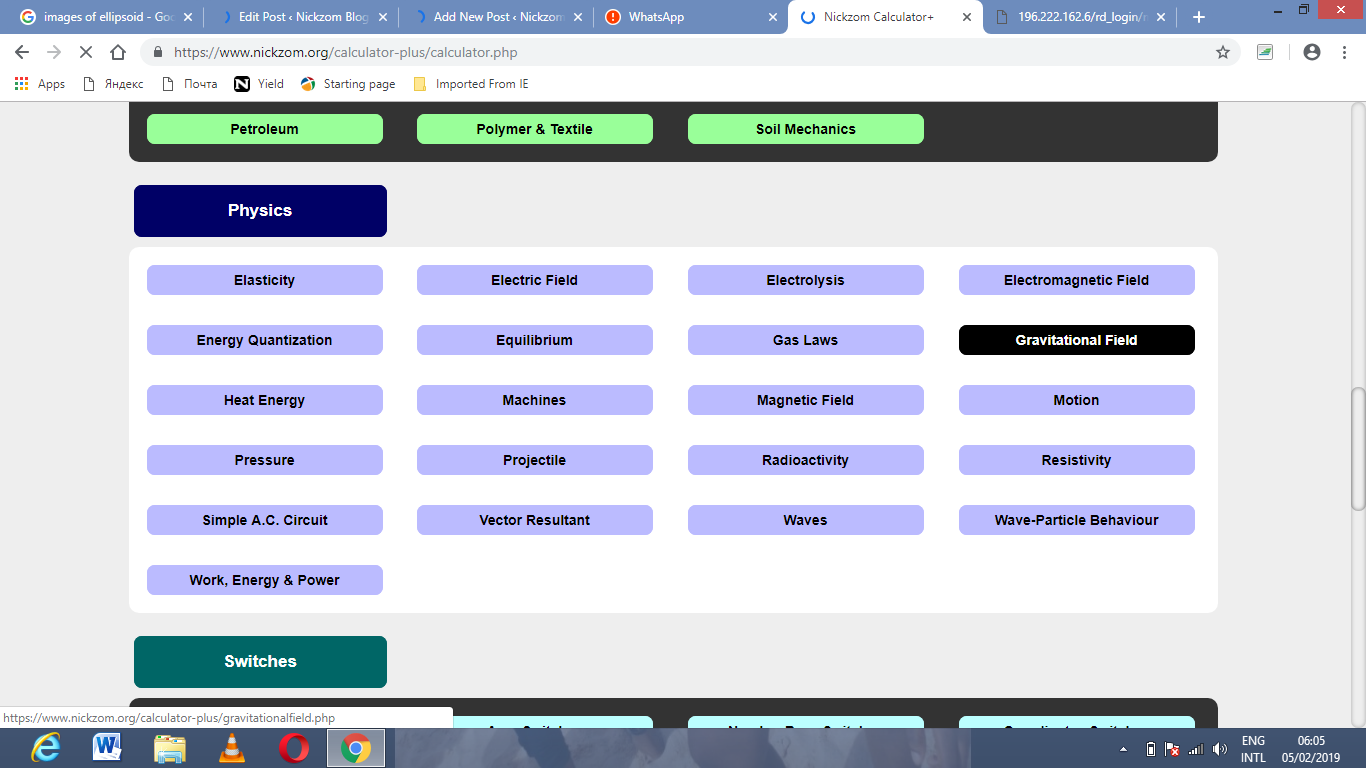Now, Click on Gravitational Force under Gravitational Field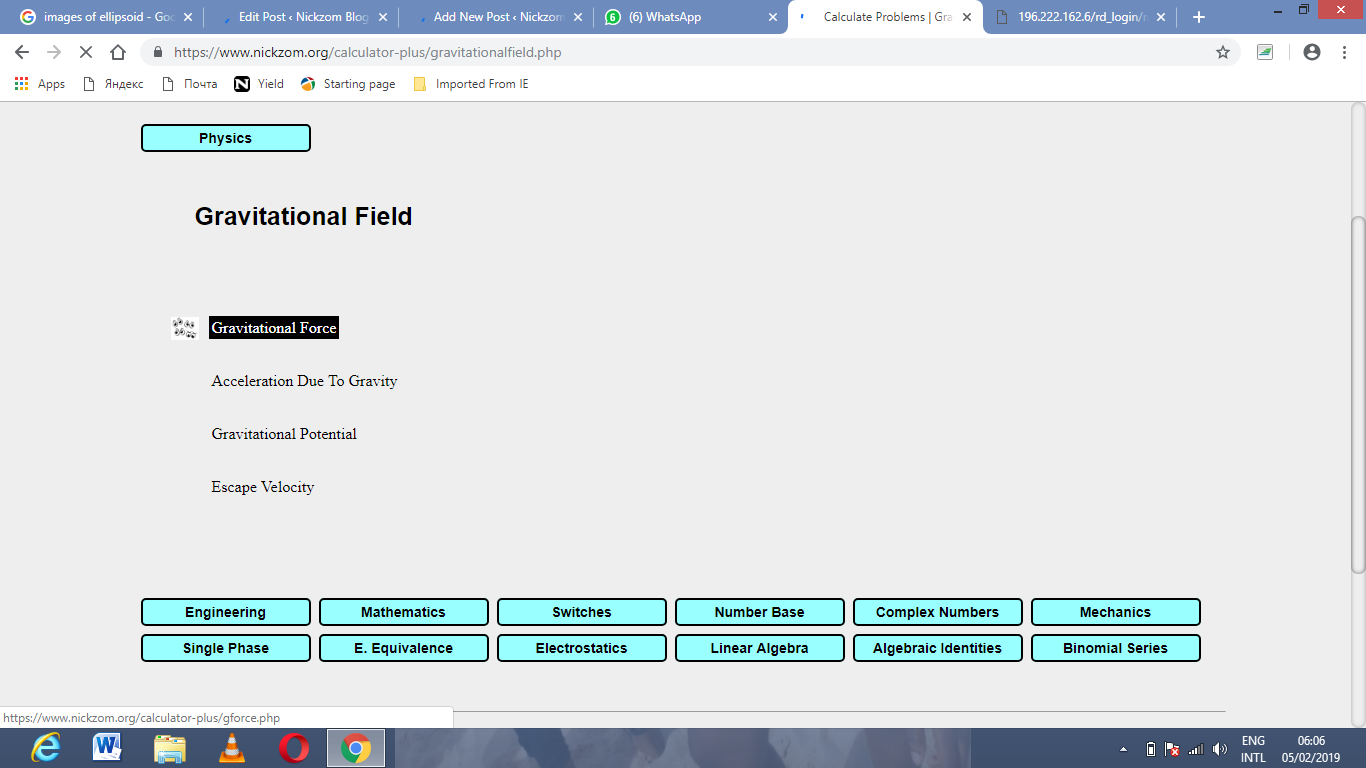The screenshot below displays the page or activity to enter your values, to get the answer for the gravitational force according to the respective parameters which are the mass (m1), mass (m2) and radius between the masses (R).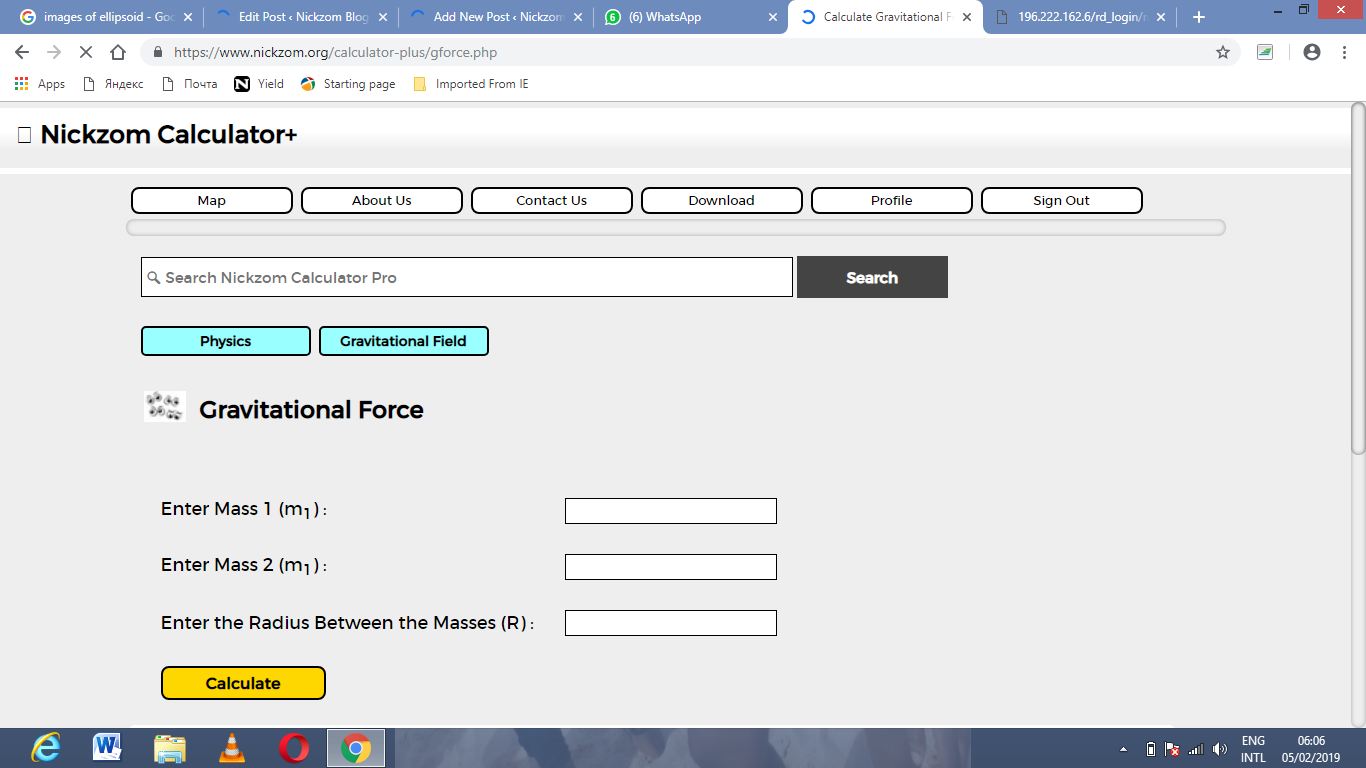Now, enter the values appropriately and accordingly for the parameters as required by the example above where the mass (m1) is 8 cm, mass (m2) is 10 cm and radius between the masses (R) is 14 cm.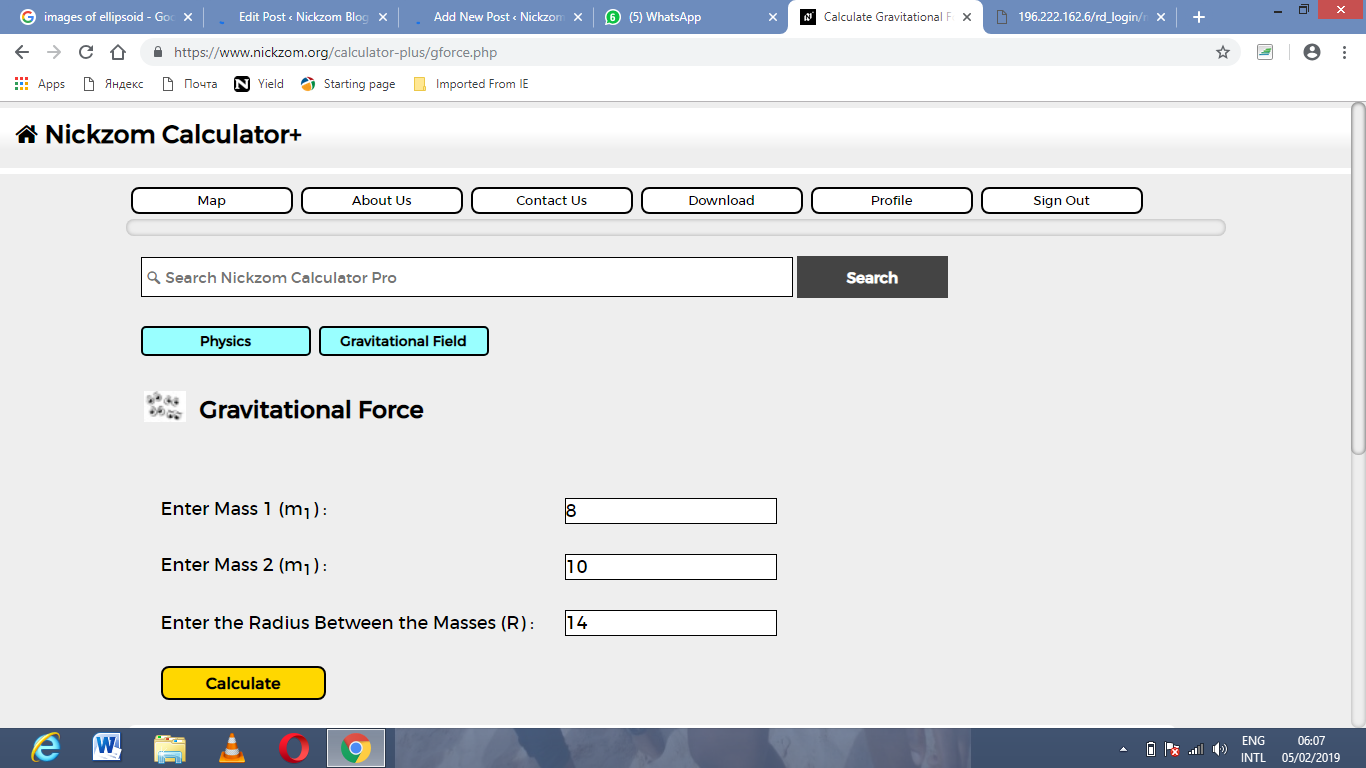Finally, Click on calculate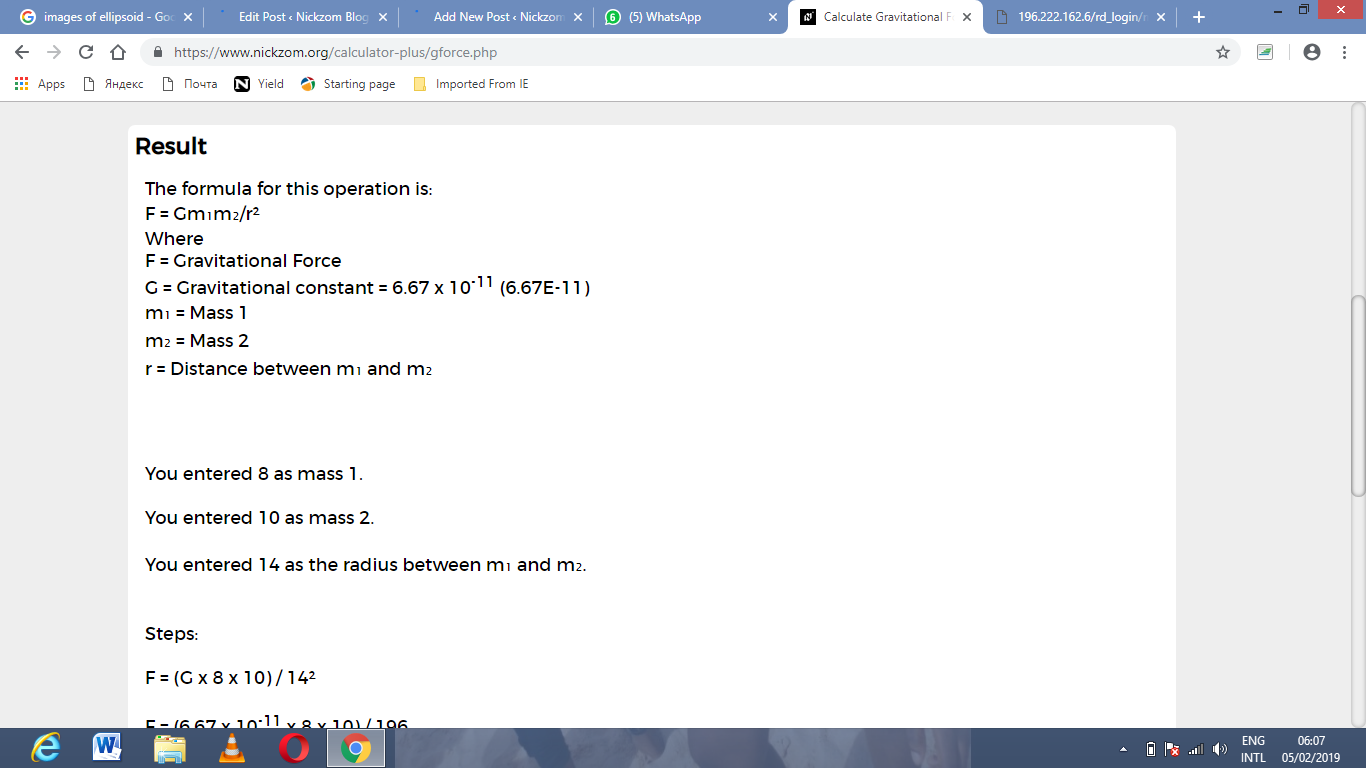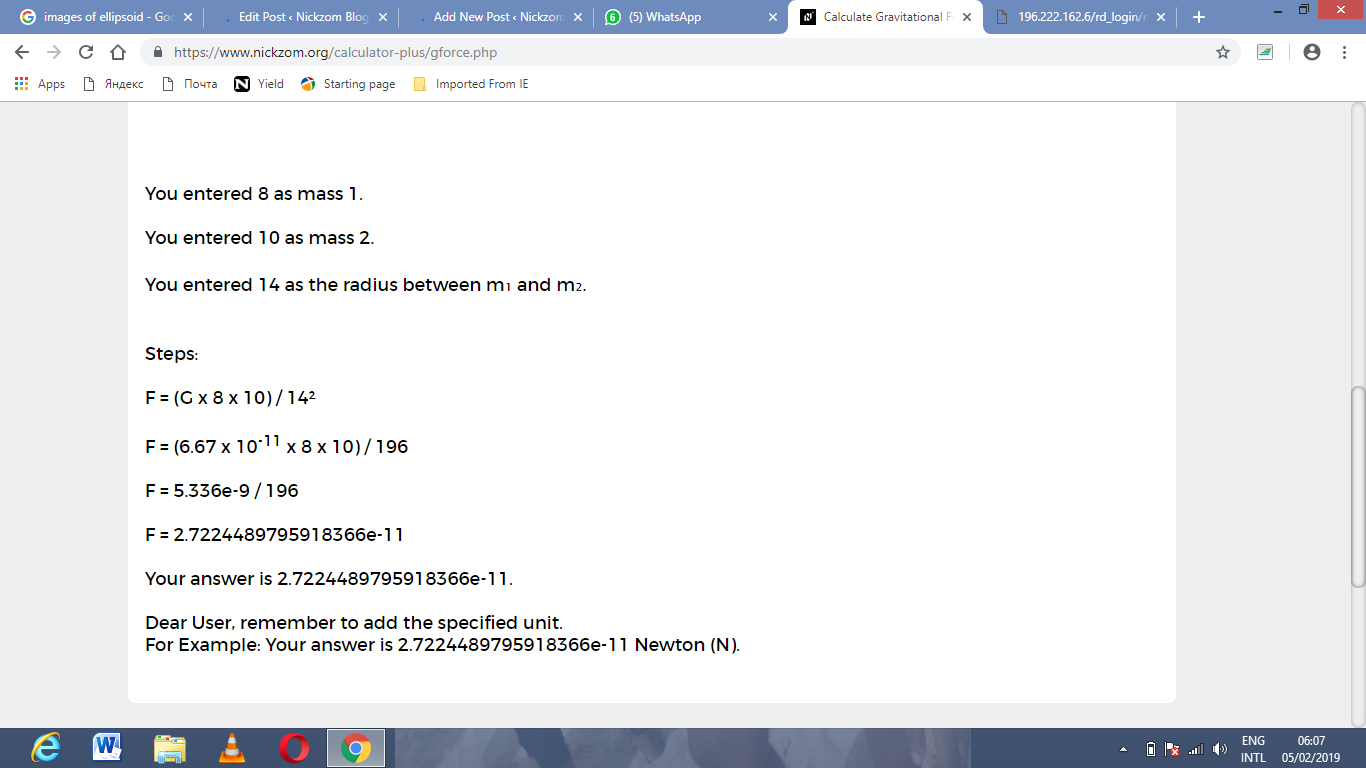As you can see from the screenshot above, Nickzom Calculator – The Calculator Encyclopedia solves for the gravitational force and presents the formula, workings and steps too.